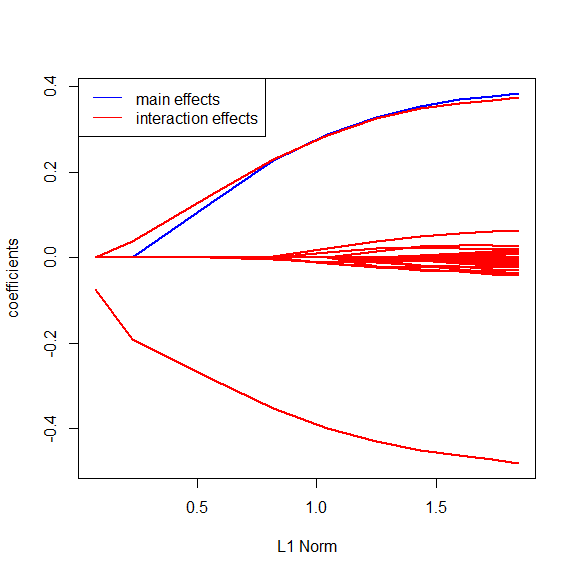# The ‘xyz’ Algorithm for Fast Interaction Search in High-Dimensional Data

The R-Package xyz contains an algorithm to estimate product interactions between a response vector $$Y$$ and predictors $$X$$. For example if all data were binary in $$\{-1,1\}$$ one would like to find the pair $$(l,k)$$ maximizing: $\begin{equation} \mathbb{P}(Y=X_l X_k). \end{equation}$ A brute force approach would cycle through all possible pairs, which implies a quadratic run-time if there are $$p$$ variables. The xyz-Algorithm provably solves this problem in sub-quadratic runt-ime. Its run-time depends on the interaction strength of the strongest pair. xyz can recover pairs in almost linerar run-time if the interaction is strong.

## Functions

The xyz package offers two functions for interaction search:

• xyz_search: If you want to search for the single pair that maximizes the probability $\begin{equation} \mathbb{P}(Y=X_l X_k) \textrm{ or } |Y^T X_l X_k| \end{equation}$ you use the function xyz_search(X,Y,L,N,binary,negative). The inputs are defined as: X: n x p design matrix. Can be either continuous or binary. Y: n dimensional response vector. Continuous or binary. L: number of projection steps. Run-time scales linear in L. N: number of closest pairs that will be returned. binary: set to true if X is binary. negative: set to true if you also want to consider interactions with a negative sign.
• xyz_regression: If you want to fit a regression model, of the form: $\begin{equation} Y_i=\beta_0 + \sum_{l=1}^p \beta_l X_{il} + \sum_{l=1}^p \sum_{k \geq l}^p \theta_{lk} X_{il} X_{ik}. \end{equation}$ The elastic net estimator puts a penalty of the form $\begin{equation} \lambda (\alpha (\|\beta\|_1+\|\theta\|_1)+(1-\alpha)(\|\beta\|_2+\|\theta\|_2)) \end{equation}$ on the parameter vectors. To fit such a model you use the function xyz_regression(X,Y,lambdas,n_lambda,alpha,L). The inputs are defined as: X: n x p design matrix. Can be either continuous or binary. Y: n dimensional continuous response vector. lambdas: user defined path (not recommended). n_lambda: Number of lambdas on path. Either n_lambda or lambdas have to be set. alpha elastic net parameter. L: number of projection steps. Run-time scales linear in L.

## Examples

xyz_search:

## Warning in normalizePath(path.expand(path), winslash, mustWork):
## path="C:/Users/rds37/AppData/Local/Temp/Rtmp6xMSEF/Rbuild39447baa666f/
## xyz/vignettes/../inst/include": The system cannot find the path specified
#set dimensions
n<-100;p<-1000;
#create data
X<-matrix(2*rbinom(n*p,1,0.5)-1,n,p)
Y<-X[,1]*X[,2]

#find top 10 interactions
result<-xyz_search(X,Y,L=5,N=10,binary=TRUE,negative=TRUE)
#the first element contains the interaction pairs the second element contains their strength
print(result)
## intereaction pair: (1,2) strength: 1
## intereaction pair: (217,398) strength: 0.46
## intereaction pair: (524,888) strength: 0.42
## intereaction pair: (685,921) strength: 0.42
## intereaction pair: (18,105) strength: 0.4
## intereaction pair: (360,945) strength: 0.38
## intereaction pair: (23,68) strength: 0.38
## intereaction pair: (405,643) strength: 0.38
## intereaction pair: (415,668) strength: 0.38
## intereaction pair: (123,554) strength: 0.38

These were now just L=10 runs. This means we discovered the interaction in about $$\mathcal{O}(np^{1.005})$$ operations instead of $$\mathcal{O}(np^2)$$.

xyz_regression:

#set dimensions
n<-100;p<-1000;
#create data
X<-matrix(rnorm(n*p),n,p)

#build model
Y<-3*X[,5]+2*X[,1]*X[,2]-3*X[,7]*X[,4]+rnorm(n)

#find top 10 interactions
result<-xyz_regression(X,Y,L=10,n_lambda=10,alpha=0.9)
#the first element contains the main effects and the third the interaction effects, we look at the fifth lambda
print(result)
## Lambda sequence:
## lambda1=0.57601
##  lambda2=0.44598
##  lambda3=0.34531
##  lambda4=0.26736
##  lambda5=0.20701
##  lambda6=0.16028
##  lambda7=0.1241
##  lambda8=0.09608
##  lambda9=0.07439
##  lambda10=0.0576
## Discovered main effects: 1 Discovered interaction effects: 42
## Model parameters:
## intercept: -4.566117e-18
## Printing effects for lambda10=0.0576
## Main effects:
## Main effect: 5 coefficient: 0.3840953
## Interaction effect:
## Interaction effect: (4,7) coefficient: -0.4812117
## Interaction effect: (1,2) coefficient: 0.3750244
## Interaction effect: (208,479) coefficient: 0.06235549
## Interaction effect: (170,203) coefficient: -0.04201977
## Interaction effect: (891,931) coefficient: -0.03821096
## Interaction effect: (104,271) coefficient: -0.03634871
## Interaction effect: (393,592) coefficient: -0.03041407
## Interaction effect: (38,610) coefficient: 0.02600682
## Interaction effect: (23,847) coefficient: -0.02076678
## Interaction effect: (570,630) coefficient: -0.02047379
## Interaction effect: (423,481) coefficient: 0.01993759
## Interaction effect: (26,450) coefficient: 0.01842155
## Interaction effect: (583,825) coefficient: -0.01752191
## Interaction effect: (7,981) coefficient: -0.01726098
## Interaction effect: (104,453) coefficient: 0.01579584
## Interaction effect: (464,798) coefficient: -0.01543339
## Interaction effect: (449,911) coefficient: -0.01507906
## Interaction effect: (305,484) coefficient: 0.01480733
## Interaction effect: (338,809) coefficient: -0.01444916
## Interaction effect: (584,681) coefficient: -0.01396515
## Interaction effect: (214,615) coefficient: -0.01351377
## Interaction effect: (329,677) coefficient: -0.01337054
## Interaction effect: (562,565) coefficient: 0.01256765
## Interaction effect: (247,392) coefficient: -0.0123604
## Interaction effect: (23,186) coefficient: -0.01199699
## Interaction effect: (20,58) coefficient: -0.01157434
## Interaction effect: (332,712) coefficient: 0.01049054
## Interaction effect: (553,659) coefficient: -0.009991351
## Interaction effect: (596,708) coefficient: -0.009258795
## Interaction effect: (11,237) coefficient: -0.009236588
## Interaction effect: (354,876) coefficient: -0.008922487
## Interaction effect: (467,499) coefficient: -0.008647326
## Interaction effect: (708,812) coefficient: 0.006859055
## Interaction effect: (236,946) coefficient: -0.005231367
## Interaction effect: (531,709) coefficient: -0.004513708
## Interaction effect: (479,822) coefficient: 0.003664453
## Interaction effect: (106,691) coefficient: 0.003388497
## Interaction effect: (77,830) coefficient: -0.002939483
## Interaction effect: (661,694) coefficient: -0.002512046
## Interaction effect: (584,675) coefficient: 0.002174015
## Interaction effect: (114,941) coefficient: -0.00206018
## Interaction effect: (106,831) coefficient: 0.001846254
plot(result)
#predict
predict(result,matrix(rnorm(10*p),10,p))
##              [,1]
##  [1,]  1.05718927
##  [2,]  0.10747538
##  [3,]  0.12871009
##  [4,] -0.66864664
##  [5,]  0.64332971
##  [6,] -0.04412215
##  [7,] -0.05661955
##  [8,] -0.19031556
##  [9,]  0.33553702
## [10,]  1.20085180